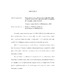## Fundamental domains for proper affine actions of Coxeter groups in three dimensionsWe study proper actions of groups $G \cong \Z/2\Z \ast \Z/2\Z \ast \Z/2\Z$ on affine space of three real dimensions. Since $G$ is nonsolvable, work of Fried and Goldman implies that it preserves a Lorentzian metric. A subgroup $\Gamma < G$ of index two acts freely, and $\R^3/\Gamma$ is a Margulis spacetime associated to a hyperbolic surface $\Sigma$. When $\Sigma$ is convex cocompact, work of Danciger, Gu{\'e}ritaud, and Kassel shows that the action of $\Gamma$ admits a polyhedral fundamental domain bounded by crooked planes. We consider under what circumstances the action of $G$ also admits a crooked fundamental domain. We show that it is possible to construct actions of $G$ that fail to admit crooked fundamental domains exactly when the extended mapping class group of $\Sigma$ fails to act transitively on the top-dimensional simplices of the arc complex of $\Sigma$. We also provide explicit descriptions of the moduli space of $G$ actions that admit crooked fundamental domains.